NextFEM Designer allows to create and analyse steel-concrete composite sections, like beams with slabs and steel-concrete columns. A step-by-step example on a composite simple frame is presented in the following.

Since version 1.4 it is possible to create composite sections in steel and concrete, natively supported by the software, allowing to consider the proper stiffness in the analysis.

Let’s take a composite frame as example, consisting of circular columns composed by a steel Ø300mm pipe, 20mm thick, filled with concrete, and an IPE 240 beam with a concrete slab, 1m wide and 20cm thick. The width of the slab must be the effective one, estimated according to composite design code rules. The materials used are steel S275 and concrete C25/30, which can be selected through the command Modify / Materials.

The setting up of sections follow the standard procedure, except for some additional specifications.

For circular columns, we start by defining a pipe section with assigned dimensions (D = 0.30m, t = 20mm) using the Edit / Sections command. Then, by double clicking on the section just created, or alternatively clicking on the Edit properties button, you can choose the shape of the section in the dialog box that appears. In this case we must select User defined in the Type tab, as per defining the characteristics of the internal column in reinforced concrete in the following Add figures mask. The input of a new section takes place with regard to the centre of gravity of the first inserted section, graphically shown by the green dot in the graph of the Sections window.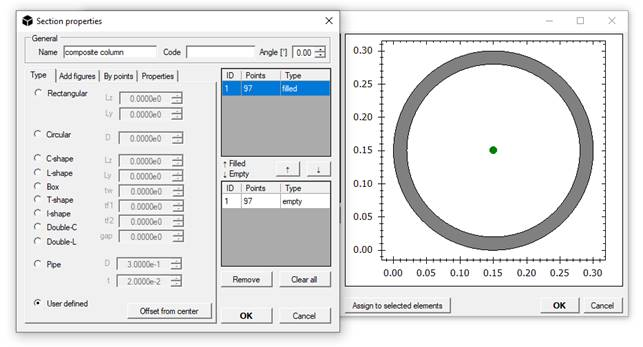The diameter D is defined equal to 0.26m, and Filled is selected as type of figure, in order to fill the previous cavity, as shown in the following figure.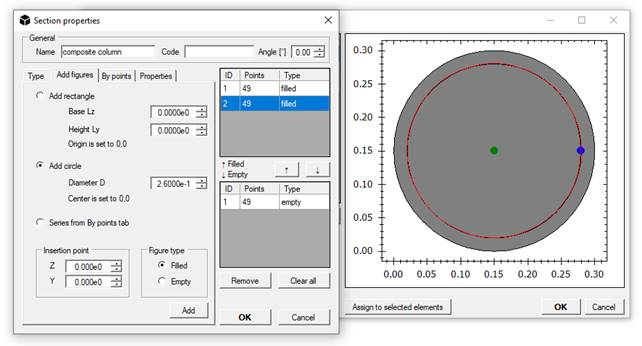Selecting the rows in the table on the right will highlight the external perimeter of the corresponding geometry in the graphic scheme, granting the user a strict control over the operations.

The material must be assigned from the By points tab, in which the coordinates of the points forming the sections previously defined are listed. It is possible to assign a material, chosen from the drop-down menu below, for each section by selecting it from the table and press the Store series button, which will save the changes.

The pipe section is defined by a solid (ID = 1, Full sections) and an empty area (ID = 1, Empty sections), consequently the steel material must also be assigned to the empty shape.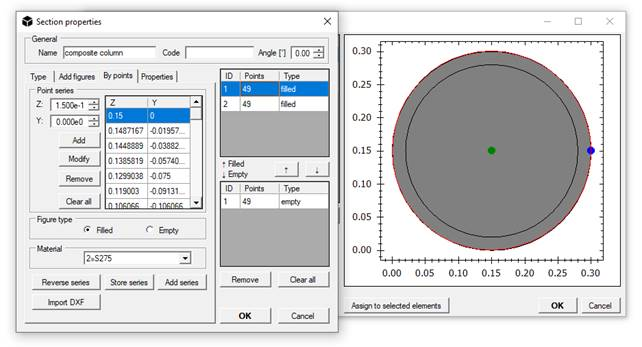Two sections are obtained, no. 1 corresponding to the steel pipe and no. 2 representing the full reinforced concrete column.

The geometric characteristics of the composite section are automatically calculated within the Properties tab, taking into account the contributions of the different materials. In accordance with Eurocode 4, the moments of inertia are normalized with respect to steel: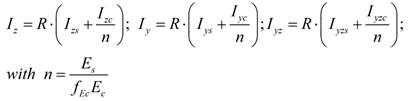R represents the reduction coefficient, while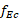is the reduction factor of the elastic modulus of concrete.

The remaining properties are calculated by dividing the contribution of concrete by the moduli ratio n. The specific properties of the element considered must be selected from the Add properties set drop-down menu, choosing Composite column and clicking the side button to input them in the data.We can proceed in the same way for the section composed by steel beam and reinforced concrete slab.

In this case, the IPE 240 shape is chosen from the built-in database, by using the Beam section drop-down menu in the Sections window. Then we add the slab by a new rectangular filled shape with the Add figures mask, with base Lz = 1m and height Ly = 0.2m. The insertion must take into account the dimensions of the steel beam, consequently the coordinates of the insertion point (represented by the lower left corner) are calculated in Z = -0.44m and Y = 0.24m with respect to the centre of gravity of the steel beam (in order to place the slab in the middle above the beam).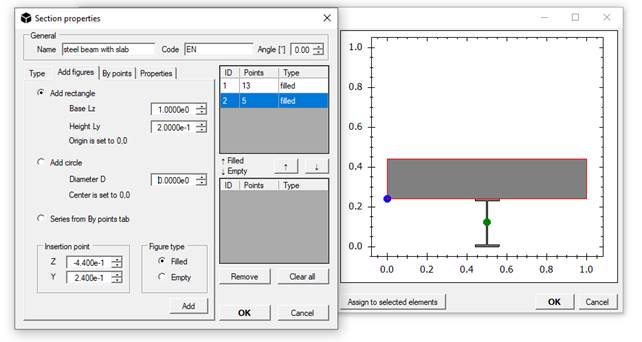As seen before, the materials are assigned from the By points tab by selecting the rows corresponding to the sections just created from the table on the right, while in this case the characteristics of the Composite Beam of Add properties set of the Properties tab must be added. The moment of inertia for negative decrease is given by the contribution of steel only, considering the section in reinforced concrete cracked, while the moment of inertia due to positive bending is given by:The overall bending stiffness is equal to the weighted sum of the positive and negative moment stiffness, as prescribed for example for seismic analysis by Eurocode 4. The positive moment of bending stiffness is calculated elastically assuming a rigid connection between steel and concrete. The negative moment of bending stiffness is instead evaluated on the basis of steel only, considering the slab as cracked.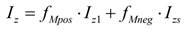The default weight values are respectively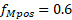and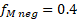.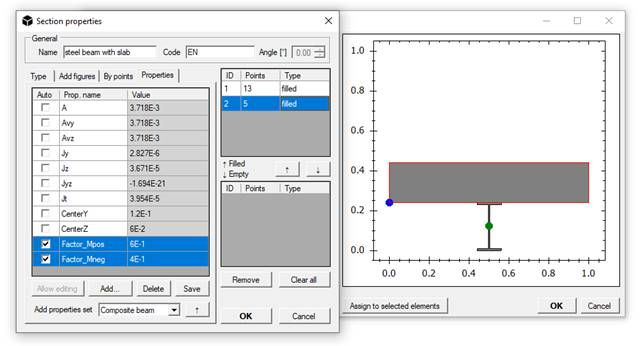The portal has a span of 5m and a height of 3m. In the modelling of column and beam elements, S275 steel is the assigned material, so that the calculation properties are correctly normalized to steel, as seen above. An error message appears if concrete (or other material) is used as base material.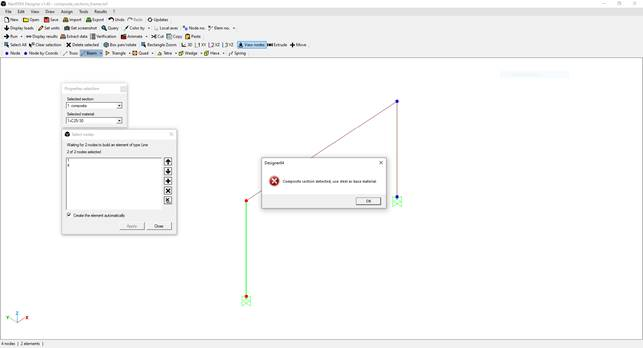Rigid restraints are applied to the base and a distributed load equal to -5 kN/m is assigned on the beam, in addition to the weight of the elements. By applying the random colouring by material, it is possible to distinguish the real composite section in each element.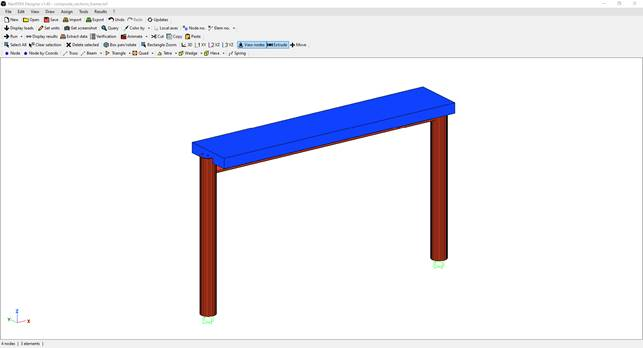The results of the analysis take into account the mechanical characteristics of the different materials composing the resistant sections, while the stress diagrams for each element remain unique.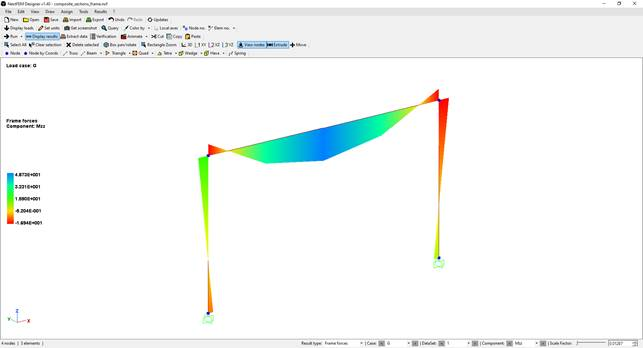Assuming for the column a reinforcement consisting of 4Ø16 longitudinal FeB44k steel, with concrete cover equal to 4cm, a resistance analysis of the composite circular section is carried out by accessing the Calculate section strength window by pressing the Strength calculator button from the Edit / Sections mask, once chosen the desired section.
For composite sections, as for those defined by the user, the insertion of reinforcement bars must be carried out by coordinates, specifying material and diameter in the appropriate selection boxes.

To enter the forces obtained from the calculation, use the Beam elements mask at the bottom right. Select the structural element from the drop-down menu (to see the number of the element on the screen press F8 or select Element number from View / View controls mask), choose the desired section of the element with the horizontal cursor and specify the load case and the instant of time (for linear analyses it is always 1, conventionally). The Get forces button imports the forces for the chosen section to perform the calculation.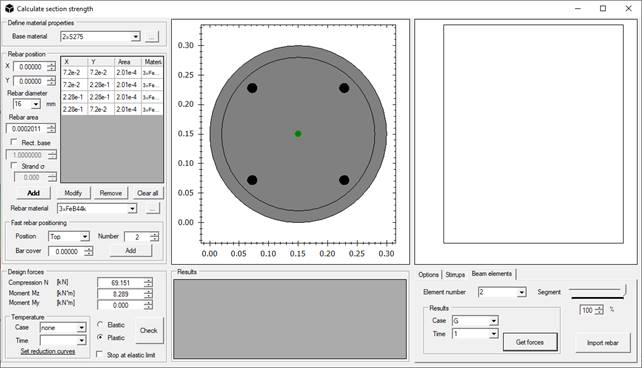In this case, design forces in column no. 2, in the base section and for load case G, are equal to N = 69.15kN and Mz = 8.29kNm. In this mask the axial force N follows an opposite convention, typical of sectional calculus: positive values indicate compression while negative values indicate tension. It is necessary to set steel as the base material (in Define material properties at the top left of the mask) for the resistant calculation of the section. After selecting the plastic calculation, the check is carried out and the position of the neutral axis and the percentage of use of the composite section are shown.The same procedure can be used for the resistant calculation of the beam section. The plastic calculation is carried out in the hypothesis of a completely rigid and resistant connection between the metal beam and the concrete slab; in other words, no sliding is allowed.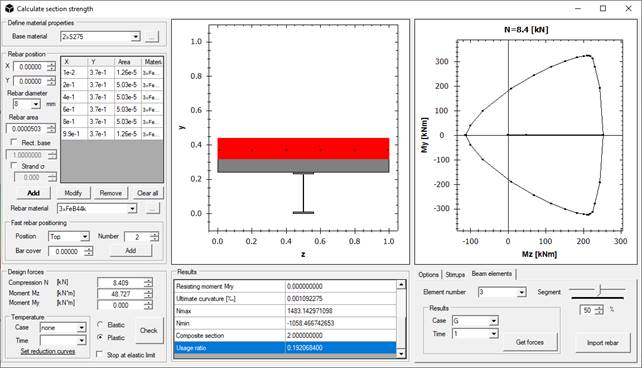As the previous image shows, the full My-Mz domain for the specified N can be obtained.

With NextFEM Designer you can easily afford the calculation of composite structures.